# Goal Seek In ExcelArticle byJeevan A Y## What Is Goal Seek In Excel?

Goal Seek is a what-if analysis tool in Excel that allow users to find the required number to achieve a targeted goal (result or value). In simple words, the Goal Seek in Excel feature helps us find the input value to obtain the targeted output automatically.

For example, let us assume that Allen wants to make a profit of \$3,000 per month by selling iron rods. So, he sells around 350 iron rods for \$3 each.

However, the revenue he can make by selling 350 iron rods is only \$1,050. So, if he has to increase his income to \$3000, he must know how many more iron rods he needs to sell. Using Goal Seek in excel, we can find the set value with the following steps:

• Go to Data -> What If Analysis -> Goal Seek.
• The Goal Seek window pops up. Enter the required values in the parameters.
• Click OK to obtain the results.

Clearly, the table implies that Allen needs to sell 1000 iron rods to earn a revenue of \$3,000.

Therefore, we can easily find the values using Goal Seek in Excel.

###### Key Takeaways
• The purpose of Goal Seek in Excel is to find the required value based on a target value.
• The formula for calculating the actual number remains the same, and the goal seek feature will only change the dependent cell.
• In addition, we can find the Goal Seek in Excel result using functions such as PMT, Future Value (FV), and Present Value (PV).
• The formula cell cannot include circular references.
• We should remember to enable iterative calculation in Excel to perform Goal Seek feature.

### Parameters of Goal Seek in Excel

Using the Goal Seek feature is simple. It is available in the Forecast group of the Data tab under the What-If Analysis drop-down list.

Goal Seek in Excel consists of 3 parameters. Therefore, in the Goal Seek window, we need to fill all three to find the value.

Set cell: We should select the excel cell reference of the target cell for which we want to calculate the value in this dialog box.

To Value: Here, we need to enter the desired output manually.

By Changing Cell: In this parameter, we have to choose the cell containing the input value, i.e., the set value.

Please Note: Using Goal Seek in Excel, we can only change one variable at a time. Therefore, we can select multiple cells to find the results using this feature.

### How To Use Goal Seek? (With Steps)

The purpose of Goal Seek in Excel is to find the required value to achieve the targeted value. For instance, Mr. Tyler is looking for a home loan of \$3,000. However, he can afford to pay the EMI amount of \$5,000 for six years.

Also, the interest rate offered by the bank is 10% P.A. Using PMT (Payment) function, we have found that Mr. Tyler has to pay an EMI amount of \$5,558 per month.

Using Goal Seek in Excel, we can find the ideal interest rate.

The steps of Goal Seek in Excel are as follows:

The steps of Goal Seek in Excel are as follows:

1. Go to the Data tab. Click on the What-If Analysis drop-down list and then, select the Goal Seek… option.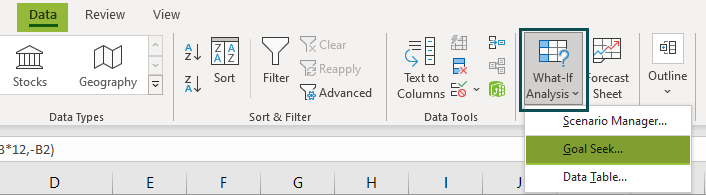2. The Goal Seek window appears in Excel.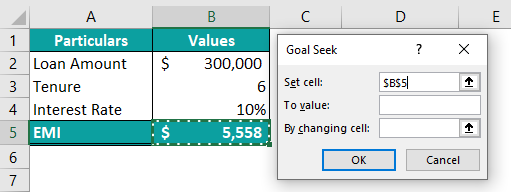We need to enter the three parameters to find the values.

3. We should select the cell reference of the target cell for which we want to calculate the value in this dialog box.

Hence, choosing cell B5.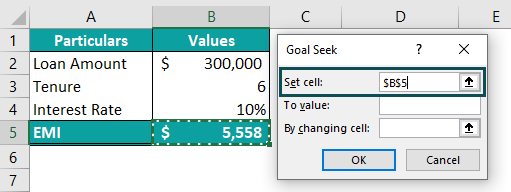4. Here, we need to enter the desired output manually. So, enter 5000 in the To Value: dialog box.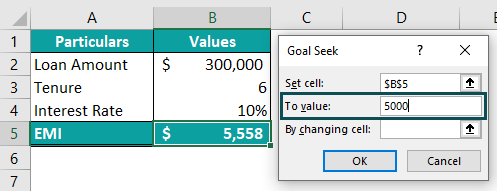5. Finally, we have to choose the input cell in the By changing cell: parameter.

Therefore, choose cell B4.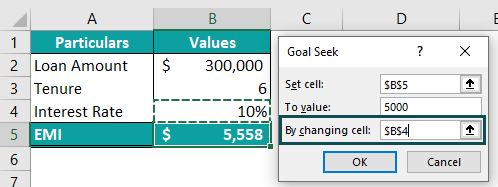6. Click OK. The Goal Seek Status window pops up with the results.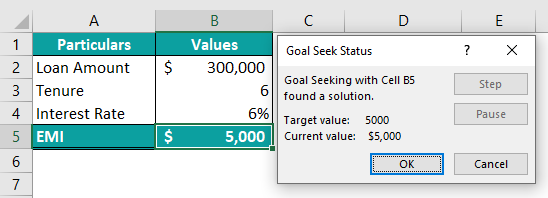From the table, it is clear that Mr. Tyler needs to bring down the interest rate from the proposed 10% to 6% to match his maximum capacity of paying EMI of \$5,000 per month.

Thus, we can use Goal Seek in Excel to obtain the results.

### Examples

Let us look at the following examples to understand the method and purpose of Goal Seek in Excel.

#### Example 1: Find the Number of Units to Reach Specific Sales Value

Mr. Felipe runs a bookstore and wants a total revenue of \$5,000 per month. The maximum number of books he can sell in a month is limited to 80. At present, he is selling his books at the rate of \$50 per book.

Step 1: First, open Goal Seek… option from What-If Analysis under the Data tab.

Step 2: In some time, the Goal Seek window pops up.

Choose cell B4 for the Set cell: dialog box.

Step 3: Next, enter the targeted sales value of \$5,000 in the To value: parameter.

Step 4: Then, select cell B3 in the By Changing Cell: box.

Step 5: Click OK. The Goal Seek Status window appears.

Now, with the returned results, it is clear that Mr. Felipe should sell each of his books at \$63 to achieve the targeted sales amount of \$5,000 in a month.

Thus, we can use Goal Seek in Excel to obtain the results.

#### Example 2: Find the ideal score to reach a specific average score

The below table lists the marks obtained by Felipe in five subjects. Using the Average Excel function, he got an average score of 71. However, he wants to score an average of 75 at the end of the last subject. We can find the ideal score using Goal Seek in Excel.

The steps of Goal Seek in Excel are as follows:

Step 1: First, click the Goal Seek… option under the Data tab.

A window named Goal Seek opens up.

Step 2: Next, choose cell B8 in the Set cell: dialog box.

Step 3: Then, set the To value: as 75.

Step 4: Now, select cell B7 in the By changing cell: parameter and click OK.

Step 5: In the Goal Seek Status pop-up window, we can see the ideal score average score of 75.

Therefore, Mr. Felipe should aim for atleast 93 in the final subject to get an average of 75.

#### Example 3: Find the monthly investment amount to reach the retirement fund

Ms. Caroline is making plans for her retirement, with a goal of \$30,00,000. She has 20 years to invest and expects a 12 percent annual return on her investment.

At present, she is planning to invest \$2,000 per month. The following is the calculation of future value based on the present investment amount.

Ms. Caroline will receive \$19,78,511 as a retirement fund if she invests \$2,000, which is far less than her aim of \$30 million.

So, she wants to know how much she needs to invest each month to accomplish her retirement goal.

The steps used to find the value using Goal Seek in Excel are as follows:

Step 1: First, select Data -> What-If Analysis -> Goal Seek… option.

Step 2: Next, we should enter the following values in the parameters and click OK.

Step 3: In some time, the Goal Seek Status window pops up with the results.

Clearly, the Goal Seek in Excel feature has returned the values that suggest that Ms. Caroline would have to invest \$3,033 per month to achieve her retirement goal of \$30 million.

This way, we can use Goal Seek to find the required value to achieve the desired goal or targeted values.

### Goal Seek not working

Though working with Goal Seek in Excel is easy, the feature may not work because of the following possible reasons:

The value cell that we are setting should contain the formula and should have the reference of the changing cell. Also, without a formula, the Goal Seek feature cannot work properly.

If so, the following error box pops up.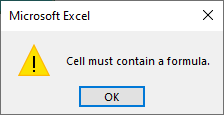Also, iteration in Excel should be turned on; otherwise, Goal Seek cannot run the iterations to arrive at the targeted value.

### Important Things To Note

• Goal Seek in Excel can change only one variable. Therefore, we cannot use the feature to change multiple cells.
• The Set cell: parameter should be a cell reference, and so, the given cell reference should contain the formula which will be impacted by the changing cell.
• By changing cell: parameter should be a cell reference and must be included as part of the formula cell.
• Also, the feature only returns the numeric value as an end output; therefore, it only requires numeric inputs.

What Does Goal Seek do in Excel?

Goal Seek helps us find the required value to achieve the targeted value. The required value is a variable, and by changing this variable, we can find the targeted value.

How to do Goal Seek in Excel?

Finding results using Goal Seek in Excel is simple.

Consider the following example.

Let us assume that Mr. Henry wants to earn a revenue of \$5,000 in a month by selling ice creams. He has 700 ice creams as stock and plans to sell each for \$6.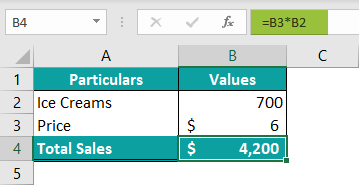However, the revenue he can make from 700 ice creams is only \$4,200. So, if he has to increase his income to \$5000, he must know how many ice creams he needs to stock. Using Goal Seek in excel, we can find the set value with the following steps:

Step 1: First, go to Data -> What If Analysis -> Goal Seek.

Step 2: The Goal Seek window pops up.

From the table, we know that

B4 is the Set Cell:
5000 is the To value:
B2 is the By changing cell: value.

So, select the values in the respective dialog boxes.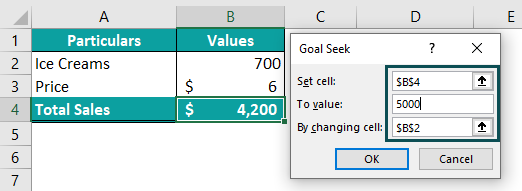Step 3: Next, click OK. We can see that the Goal Seek in excel feature has returned the required value.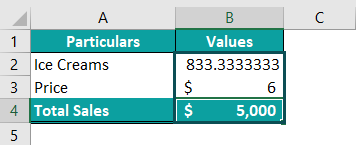Clearly, it implies that Mr. Henry needs a stock of approximately 833 ice creams to earn a revenue of \$5,000.

Therefore, we can find the values using Goal Seek in Excel with ease.

Where can I find Goal Seek in Excel?

We can enable the feature using the following steps:

Step 1: To begin with, choose the Data tab under the drop-down list.

Step 2: Next, click on the What If Analysis option from the Forecast group.

Step 3: Then, select Goal Seek… option from the drop-down list.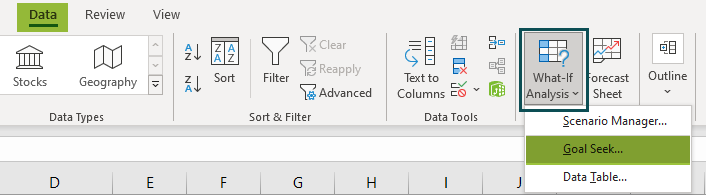Alternatively, we can automate Goal Seek in Excel using VBA macro coding.